# 传统方法

User guide: contents — metric-learn 0.6.2 documentation

# 深度学习

## 基于正负样本对的方法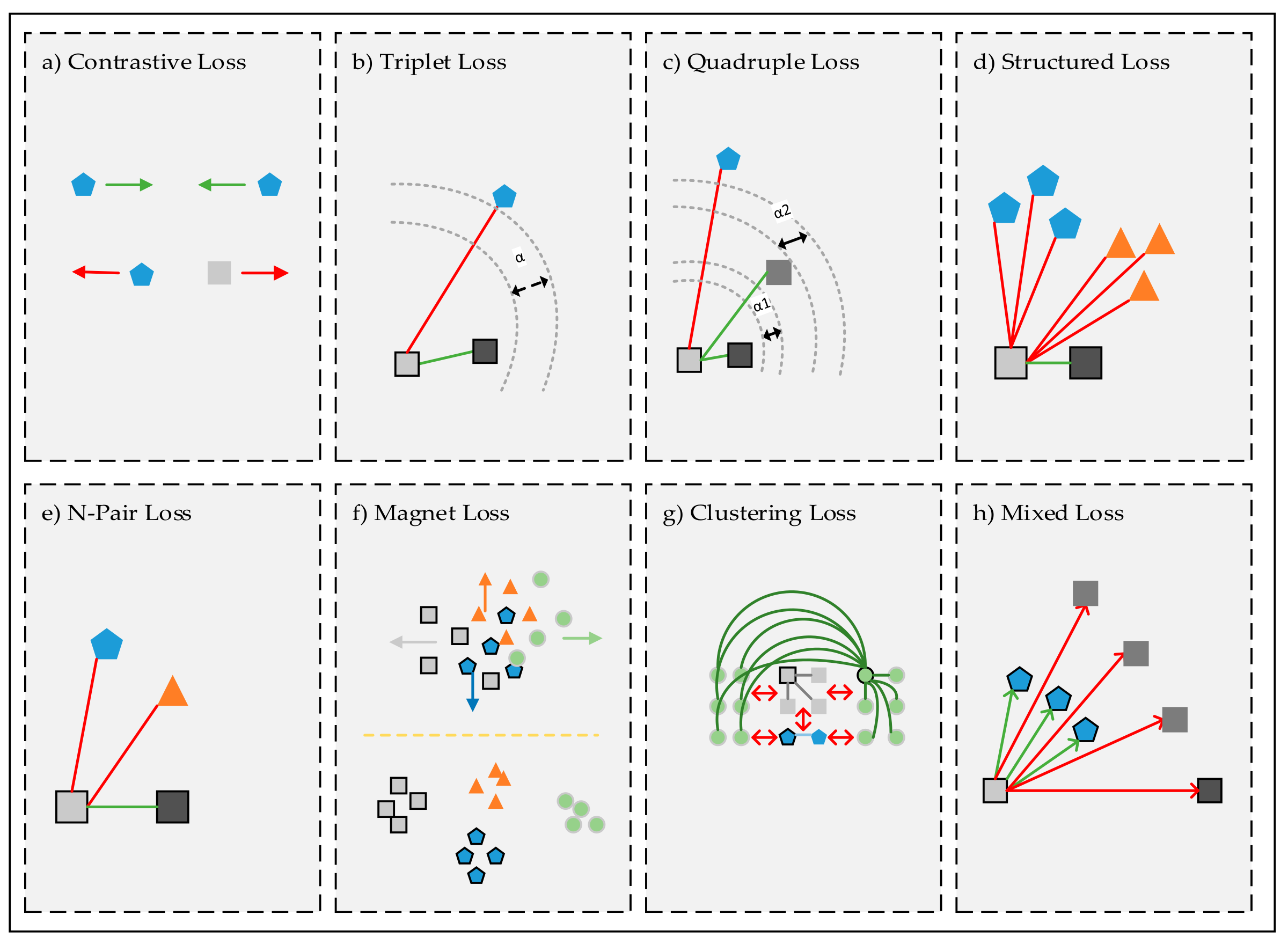### Contrastive Loss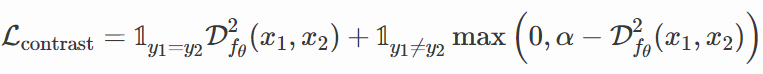### Triplet Loss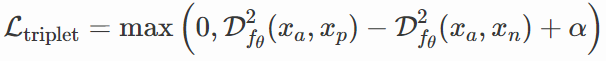xa 称为 anchor 样本，xp 为正样本，xn 为负样本。这里的 margin 同样有避免模型将所有样本映射到特征空间中同一个点的作用。同时 Triplet Loss 的一个关键点是负例挖掘（Negative Samples Mining），将 anchor 样本与正样本间的距离尽可能限制到0附近，同时将 anchor 样本与负样本的距离推开至 margin 左右，

The only requirement is that given two positive examples of the same class and one negative example, the negative should be farther away than the positive by some margin.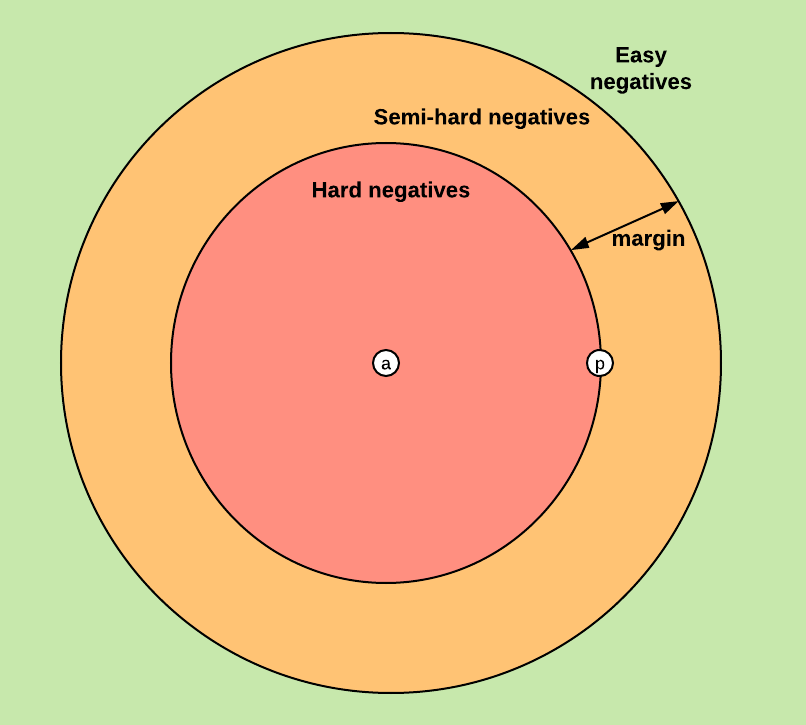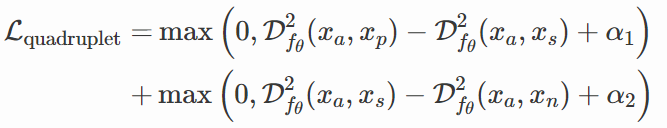### Structured Loss

Triplet Loss 中只考虑了一个负例，而忽略了其他负例。与 Triplet Loss 不同，Structured Loss 考虑的是 batch 中所有的正样本对，以及距离正样本对中两个点最近的负样本：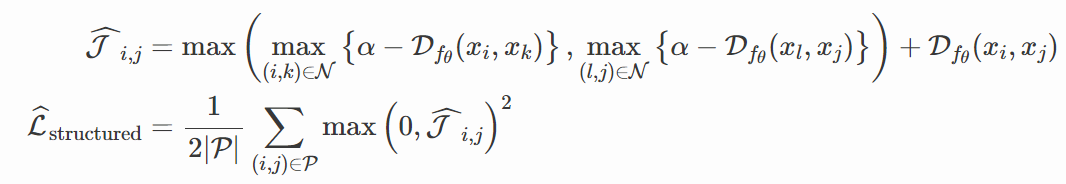N-Pair Loss 也是考虑到 Triplet Loss 中的这个缺点而提出的，只不过处理方法与 Structured Loss 不同。

## 基于交叉熵的方法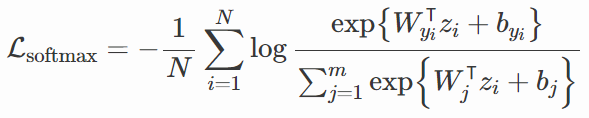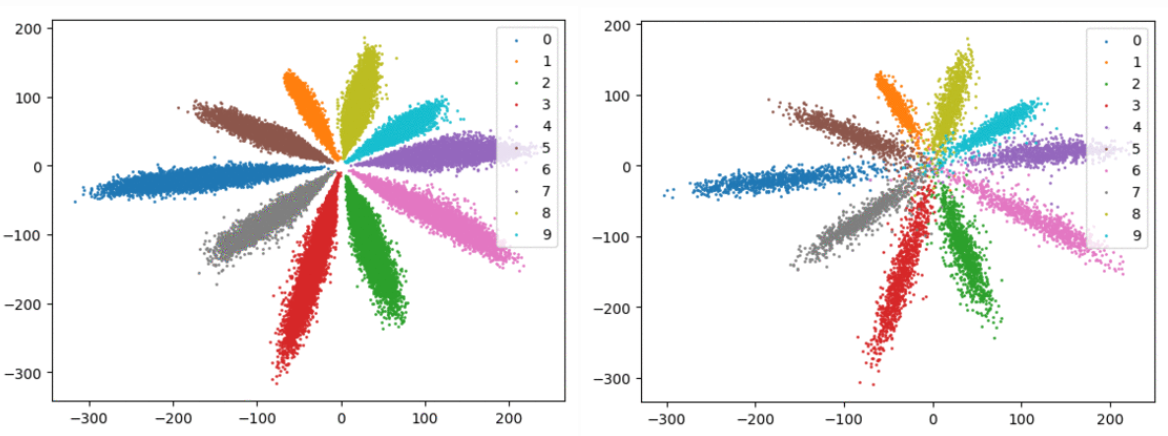### Center Loss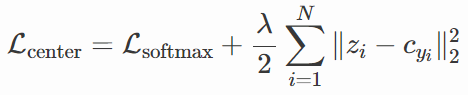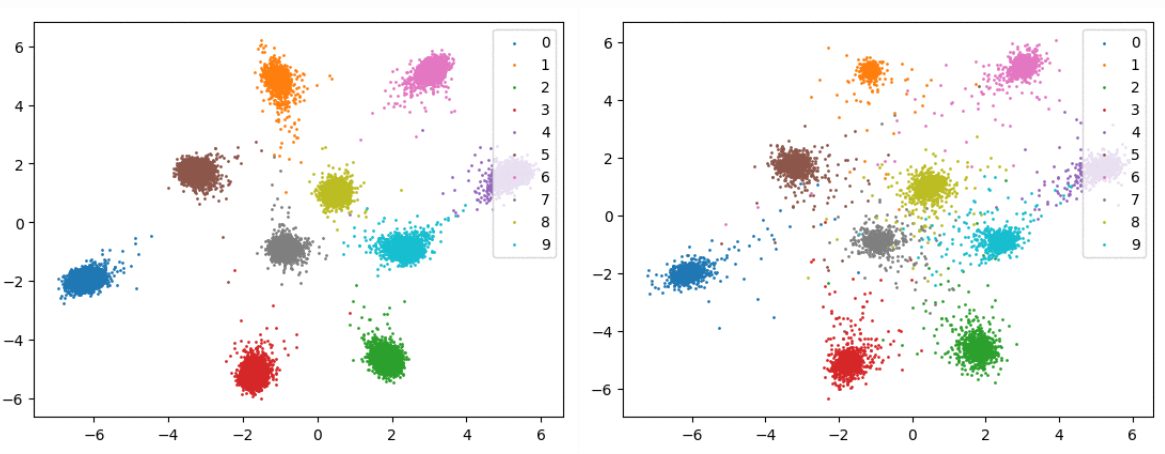### SphereFace

Center Loss 的问题是，我们不能预先知道数据集中各类别聚类中心在特征空间内是相互远离的，如果他们很靠近的话，这个正则项就起不了多大的作用。但是如果我们将每个类别的聚类中心放到距离特征空间“原点”相同的距离，即将聚类中心都映射到一个“圆”上，只要这个“圆”的半径够大，理论上我们就可以把各类别的聚类中心“推”的更加分散。这个“圆”其实就是一个超平面。这也就是 SphereFace 的做法，它是由 Softmax Loss 一步步进化而来：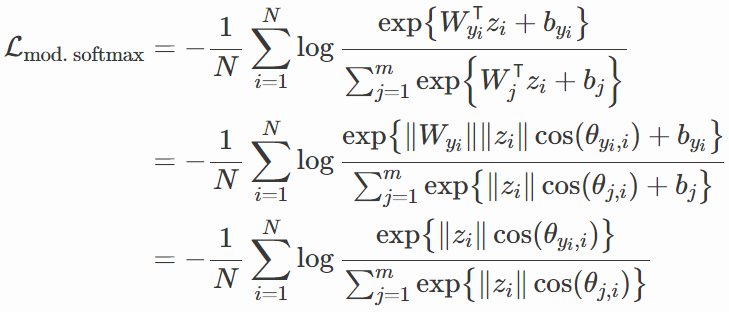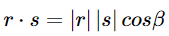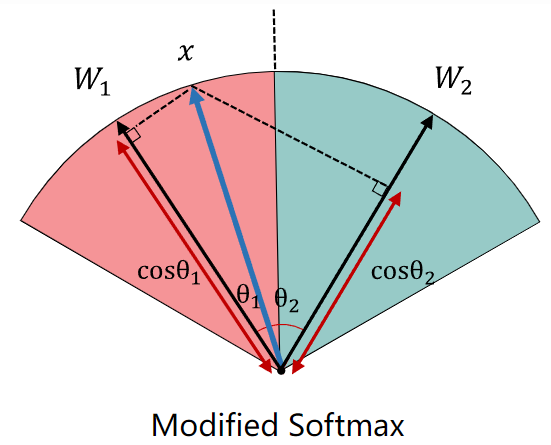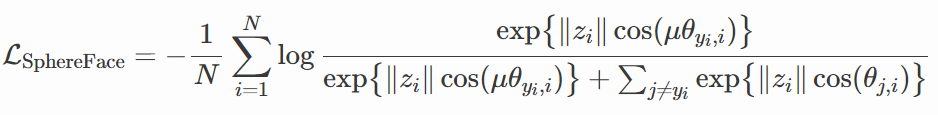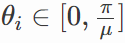SphereFace 开创了用角度距离来完成分类的先河，接下来的几种方法都是基于此提出。

### CosFace

CosFace 的做法是将特征向量 z 也进行正则化，同时加上两个超参数 s 和 m：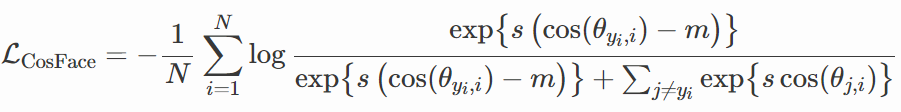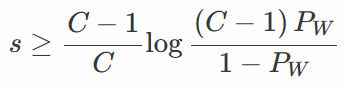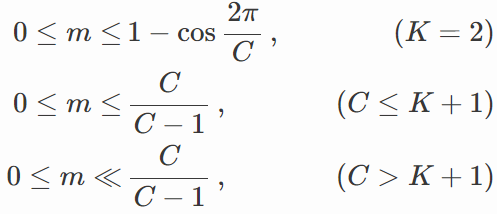### ArcFace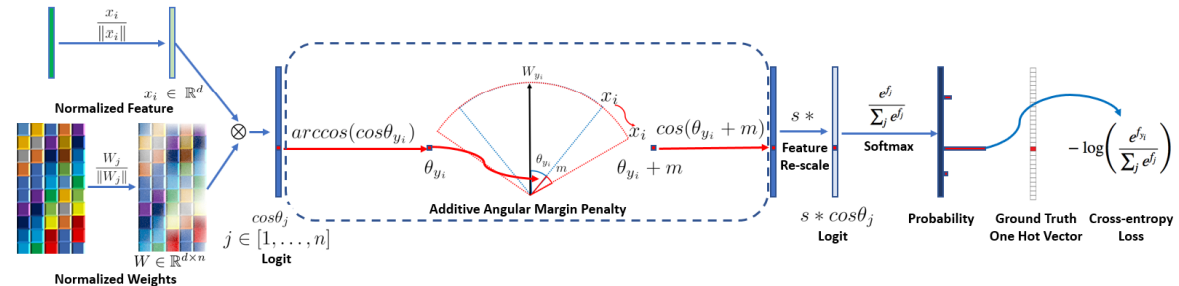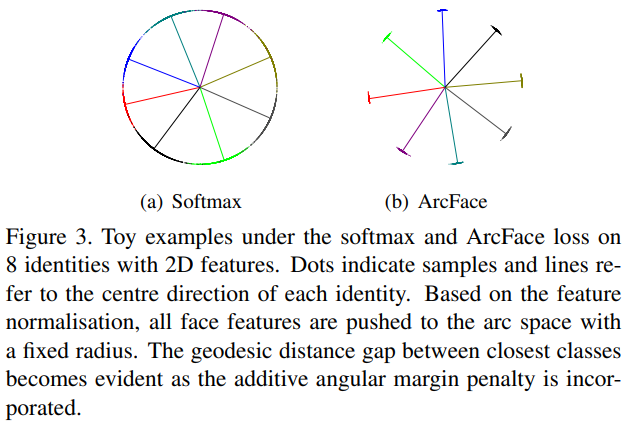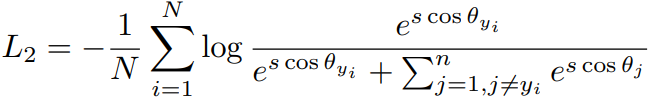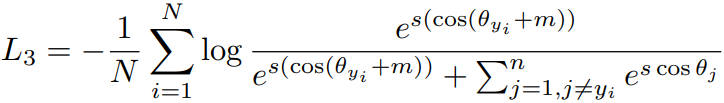``````class ArcMarginProduct(nn.Module):
r"""Implement of large margin arc distance: :
Args:
in_features: size of each input sample
out_features: size of each output sample
s: norm of input feature
m: margin
cos(theta + m)
"""
def __init__(self, in_features, out_features, s=30.0,
m=0.30, easy_margin=False, ls_eps=0.0, device="cpu"):
super(ArcMarginProduct, self).__init__()
self.in_features = in_features
self.out_features = out_features
self.s = s
self.m = m
self.ls_eps = ls_eps  # label smoothing
self.weight = nn.Parameter(torch.FloatTensor(out_features, in_features))
nn.init.xavier_uniform_(self.weight)

self.easy_margin = easy_margin
self.cos_m = math.cos(m)
self.sin_m = math.sin(m)
self.th = math.cos(math.pi - m)
self.mm = math.sin(math.pi - m) * m

self.device = device

def forward(self, input, label):
# --------------------------- cos(theta) & phi(theta) ---------------------
cosine = F.linear(F.normalize(input), F.normalize(self.weight)) # F.normalize(input)、F.normalize(self.weight) 是公式中对输入和权重的正则化
sine = torch.sqrt(1.0 - torch.pow(cosine, 2))
phi = cosine * self.cos_m - sine * self.sin_m    # 三角公式
if self.easy_margin:
phi = torch.where(cosine > 0, phi, cosine)
else:
phi = torch.where(cosine > self.th, phi, cosine - self.mm)
# --------------------------- convert label to one-hot ---------------------
# one_hot = torch.zeros(cosine.size(), requires_grad=True, device='cuda')
# one_hot = torch.zeros(cosine.size(), device=self.device)
one_hot = torch.zeros_like(cosine)
one_hot.scatter_(1, label.view(-1, 1).long(), 1)
if self.ls_eps > 0:
one_hot = (1 - self.ls_eps) * one_hot + self.ls_eps / self.out_features
# -------------torch.where(out_i = {x_i if condition_i else y_i) ------------
output = (one_hot * phi) + ((1.0 - one_hot) * cosine)
output *= self.s

return output``````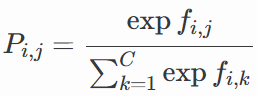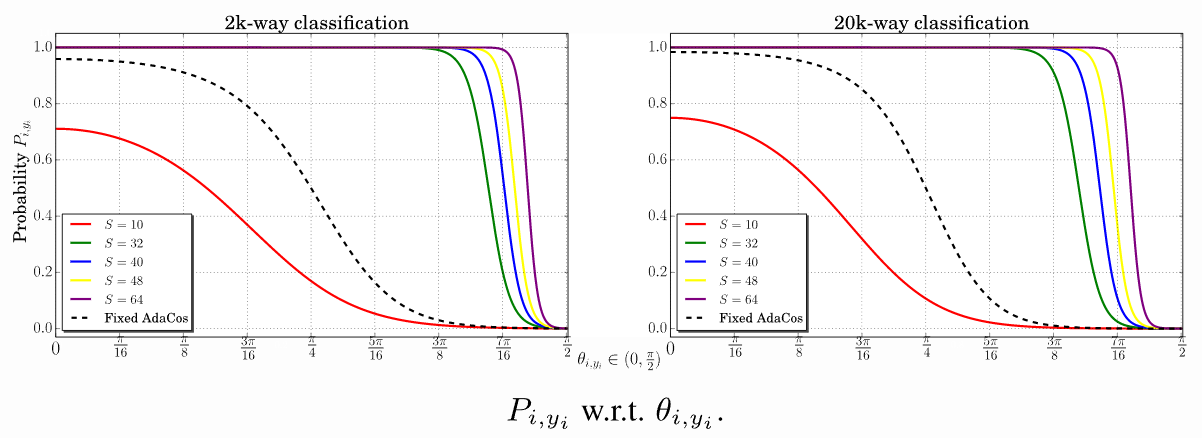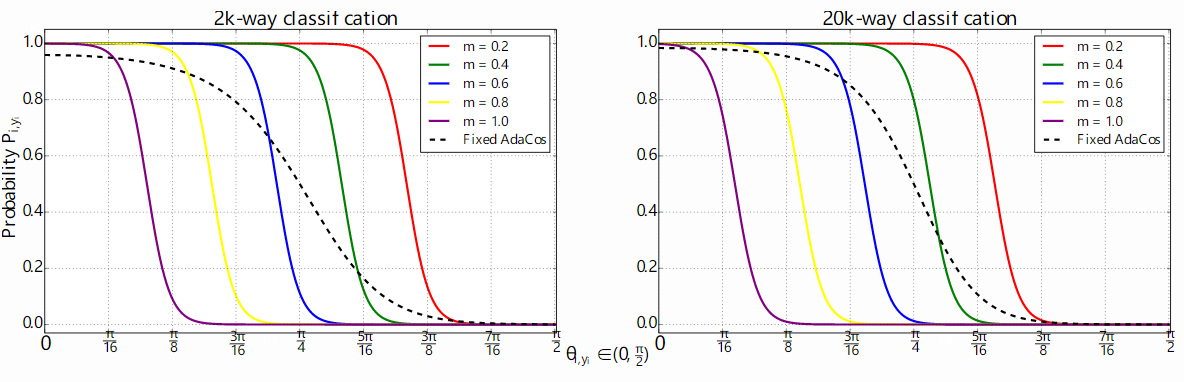### Sub-center ArcFace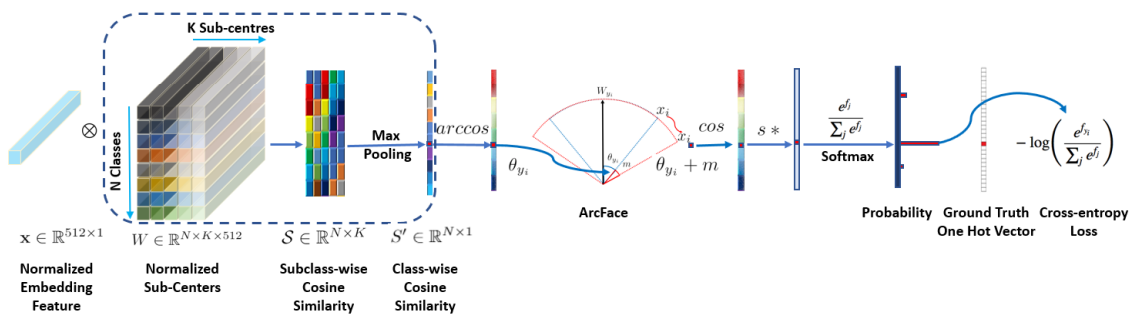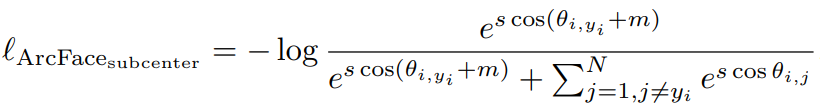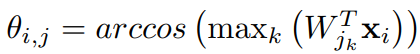``````class ArcMarginProduct_subcenter(nn.Module):
def __init__(self, in_features, out_features, k):
super().__init__()
self.weight = nn.Parameter(torch.FloatTensor(out_features * k, in_features))
self.reset_parameters()
self.k = k
self.out_features = out_features

def reset_parameters(self):
stdv = 1. / math.sqrt(self.weight.size(1))
self.weight.data.uniform_(-stdv, stdv)

def forward(self, features):
cosine_all = F.linear(F.normalize(features), F.normalize(self.weight))
cosine_all = cosine_all.view(-1, self.out_features, self.k)
cosine, _ = torch.max(cosine_all, dim=2)
return cosine``````

``````class ArcFaceLossAdaptiveMargin(nn.modules.Module):
def __init__(self, margins, out_dim, s):
super().__init__()
self.crit = DenseCrossEntropy()
self.s = s
self.register_buffer('margins', torch.tensor(margins, device="cuda:0"))
self.out_dim = out_dim

def forward(self, logits, labels):
# ms = []
# ms = self.margins[labels.cpu().numpy()]
ms = self.margins[labels]
cos_m = torch.cos(ms)  # torch.from_numpy(np.cos(ms)).float().cuda()
sin_m = torch.sin(ms)  # torch.from_numpy(np.sin(ms)).float().cuda()
th = torch.cos(math.pi - ms)  # torch.from_numpy(np.cos(math.pi - ms)).float().cuda()
mm = torch.sin(math.pi - ms) * ms  # torch.from_numpy(np.sin(math.pi - ms) * ms).float().cuda()
labels = F.one_hot(labels, self.out_dim)
labels = labels.half() if CFG.MIXED_PRECISION else labels.float()
cosine = logits
sine = torch.sqrt(1.0 - cosine * cosine)
phi = cosine * cos_m.view(-1, 1) - sine * sin_m.view(-1, 1)
phi = torch.where(cosine > th.view(-1, 1), phi, cosine - mm.view(-1, 1))
output = (labels * phi) + ((1.0 - labels) * cosine)
output *= self.s
loss = self.crit(output, labels)
return loss``````

### ArcFace with Dynamic Margin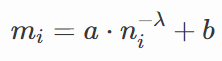## 参考

Deep Metric Learning: a (Long) Survey – Chan Kha Vu

### gmpy2的安装（python）_oooooooohhhhh的博客-程序员宅基地_gmpy2

Gmpy2 安装：（三个方法）直接再pycharm里面安装——file——setting——python interpreter—“+”搜索gmpy2安装（这个步骤可能有点点小问题，我凭记忆来的，安装模板的位置应该都能找到，就不要纠结这个小错误了）。但是我用这个安装报错，使用的下面一个方法安装的 Win+R 打开windows命令窗口，使用pip install gmpy2安装成功 若使用这个pip直接安装不成功，https://www.lfd.uci.edu/~gohlke/pythonlibs/

### EasyPlayer播放器浏览器ActiveX/OCX插件RTSP播放/抓拍/录像功能调用说明_xiejiashu的博客-程序员宅基地

EasyPlayerPro与EasyPlayer-RTSP新增ocx多窗口播放功能这里以EasyPlayerPro为例,使用方法如下:打开播放器文件夹,进入Bin/C++目录,可以看到reg.bat这个文件,以管理员身份运行 成功运行程序后,找到ocx mutiplayer.html文件,右键选择打开方式,使用ie浏览器打开ie浏览器会弹窗阻止javascript程序运行,看不到视...

### html5+ barcode_scan-二维码扫描_weixin_41961749的博客-程序员宅基地

Hello H5+ barcodeBarcode模块管理条码扫描，提供常见的条码（二维码及一维码）的扫描识别功能，可调用设备的摄像头对条码图片扫描进行数据输入。通过plus.barcode可获取条码码管理对象。常量：QR: 条码类型常量，QR二维码，数值为0EAN13: 条码类型常量，EAN一维条形码码标准版，数值为1EAN8: 条码类型常量，ENA一维条形码简版，数值为2A...

### kali linux安装后常用软件安装介绍和部分错误解决_5t4rk的博客-程序员宅基地

kali linux 安装之后整理《kaili-linux 虚拟机详细安装简本中文版》http://goo.gl/XjLs1G【普及帖】kali linux 安装之后整理By sIyuAn ps 网上收集、自己整理一些，想安装啥自己节选也不定要全部都弄1.更新软件源：root权限：leafpad /etc/apt/sources.list 用 # 注释官方源，然后添加国

### js获取 本周,本月,本季度,本年,上月,上周,上季度,去年_雨泽的博客-程序员宅基地

@author  YHC转载必须在文章第一行注明来源地址:  http://blog.csdn.net/yhc13429826359/article/details/8085641/** * 针对Ext的工具类 */var MrYangUtil=function(){ /*** * 获得当前时间 */ this.getCurrentDate=function(){ retur

### 2018VR眼镜，今天做了一个测评！_A556636928的博客-程序员宅基地

Vr眼镜是什么？近几年炒的非常火热的vr虚拟现实备受大家关注，各大科技公司也开始投产研发，推出新一代的vr眼镜，它到底是个什么东西？对我们的现实生活影响大不大？虚拟现实技术是一种可以创建和体验虚拟世界的计算机仿真系统它利用计算机生成一种模拟环境是一种多源信息融合的交互式的三维动态视景和实体行为的系统仿真使用户沉浸到该环境中。VR眼镜是什么？vr眼镜的分类外接式头戴设备用户体验较好，具备...